# To make a function call-by-reference, instead of passing variable itself, pass the address of variable. The address of the variable can be taken by using the & operator. Dereferencing is achieved in C by using the pointer (*) notation. Functions may be recursive that is a function may call itself.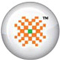PRO DATA DOCTOR it | es | pt | fr | de | jp | kr | cn | ru | nl | gr

Call-by-reference

To make a function call-by-reference, instead of passing the variable itself, pass the address of the variable. The address of the variable can be taken by using the & operator. The following calls a swap function passing the address of variables instead of the actual values.

swap(&x, &y);

Dereferencing

The problem we have now is that the function swap has been passed the address rather than the variable, so we need to dereference the variables so that we are looking at the actual values rather than the addresses of the variables in order to swap them.

Dereferencing is achieved in C by using the pointer (*) notation. In simple terms, this means placing a * before each variable before using it in order that it refers to the value of the variable rather than its address. The following program illustrates passing-by-reference to swap two values.

#include <stdio.h>
void swap(int *x, int *y);

int main()
{
int x=6, y=10;
printf("Before the function swap, x = %d and y =
%d\n\n", x, y);

swap(&x, &y);

printf("After the function swap, x = %d and y =
%d\n\n", x, y);
return 0;
}

void swap(int *x, int *y)
{
int temp = *x;
*x = *y;
*y = temp;
}

Let us see the output of the program:

 Before the function swap, x = 6 and y = 10 After the function swap, x = 10 and y = 6

Functions may be recursive that is a function may call itself. Each call to itself requires that the current state of the function is pushed onto the stack. It is important to remember this fact as it is easy to create a stack overflow, i.e. the stack has run out of space to place any more data.

The following example calculates the Factorial of a number using recursion. A factorial is a number multiplied by every other integer below itself, down to 1. For example, the factorial of the number 6 is:

Factorial 6 = 6 * 5 * 4 * 3 * 2 * 1

Therefore the factorial of 6 is 720. It can be seen from the above example that factorial 6 = 6 * factorial 5. Similarly, factorial 5 = 5 * factorial 4, and so on.

The following is the general rule for calculating factorial numbers.

factorial(n) = n * factorial(n-1)

The above rule terminates when n = 1, as the factorial of 1 is 1. Let us try to better understand it with the help of example:

#include <stdio.h>
long int factorial(int num);

int main()
{
int num;
long int f;

printf("Enter a number: ");
scanf("%d", &num);

f = factorial(num);

printf("factorial of %d is %ld\n", num, f);
return 0;
}

long int factorial(int num)
{
if (num == 1)
return 1;

else
return num * factorial(num-1);
}

Let us see the output of the execution of this program:

 Enter a number: 7 factorial of 7 is 5040

Sample Chapters from book DATA RECOVERY WITH AND WITHOUT PROGRAMMING by Author Tarun Tyagi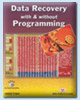Publishers of the Book Number of Pages ISBN Price of the Book BPB Publications, New Delhi, India 540 81-7656-922-4 \$69.00 (Including Shipping Charges, Cost of Book and Other expenses, Free Source Code CD included with the Book)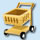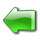page 1 | 2 | 3 | 4 | 5 | 6 | 7 | 8 | 9 | 10 | 11 | 12 | 13 | 14 | 15 | 16 | 17 | 18 | 19 | 20

 page 21 | 22 | 23 | 24 | 25 | 26 | 27 | 28 | 29 | 30 | 31 | 32 | 33 | 34 | 35 | 36 | 37

 page 38 | 39 | 40 | 41 | 42 | 43 | 44 | 45 | 46 | 47 | 48 | 49 | 50 | 51 | 52 | 53 | 54

 page 55 | 56 | 57 | 58 | 59 | 60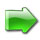© Copyright 2002-2005 DataDoctor.Biz
 Website Data Recovery | recuperación de Datos | Récupération de données | Datenrettung | Recupero dati | データ復旧 | 데이터 복구 | 数据恢复 | Восстановление данных | De terugwinning van gegevens | Ανάκτηση δεδομένων Sitemap Site map1 2 3 4 | Spanish1 2 3 | French1 2 3 | German1 2 3 | Italian1 2 3 | Portuguese1 2 3 | Japanese1 2 3 | Korean1 2 3 | Chinese1 2 3 | Russian1 2 3 | Dutch1 2 3 | Greek1 2 3 Data Recovery Book English | Spanish | French | German | Italian | Portuguese | Japanese | Korean | Chinese | Russian | Dutch | Greek